# SDR VS. DDR and Allowable Clocking Frequencies for the Digital Pattern Instrument

## 개요

With NI-Digital Pattern Driver 18.0, the minimum pulse width was reduced from 5.0 ns to 3.75 ns. This corresponds to a change from 1/5.0 ns = 200 Mbps to 1/3.75 ns = 266 Mbps. Vectors will still only be able to run with a maximum vector rate of 100 Mvectors/s, but there are additional edges that can allow you to get higher rates.

## Clocking methods (SDR vs. DDR and Allowable Clocking Frequencies)

As shown in Figure 1, there are two common ways of clocking data in applications: single data rate (SDR) and double data rate (DDR). In SDR, data is only clocked on one edge of the clock (either the rising or falling edge). This means that for SDR to have data being transmitted at X Mbps, the clock bit rate needs to be 2X Mbps. While for DDR, data is transferred on both the rising and falling edge of the clock. This means that data being transmitted at X Mbps only requires the clock bit rate to be X Mbps.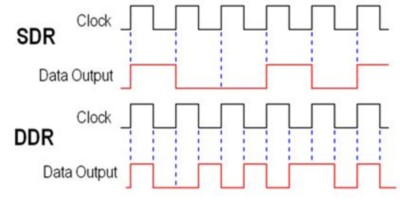Figure 1

### Clocking between 100 MHz -> 200 MHz

#### 100 MHz ≤ (f) ≤ 133 MHz

With NI-Digital Pattern Driver 18.0, the minimum pulse width is 3.75 ns, rather than 5 ns. This allows speeds up to 133 MHz at any duty cycle. The digital pattern instrument can technically get shorter pulse widths than 3.75 ns, but any values within that range are not warranted specs. If you need to go into this range, make sure to know and understand the rules below.

#### 133 MHz < (f) ≤ 160 MHz

The digital pattern instrument can generate in this range, but the signal will not have a 50% duty cycle. One of the pulse widths must be 2.5 ns. This means that any signal generated in this range will have a duty cycle distortion of between 40%/60% and 33%/67%.

Example Calculation:
If 140 MHz is the desired frequency (f), what is my duty cycle?
Known equation: 2.5ns + x = 1/f
1/140 MHz = 7.14 ns
x = 4.64 ns
Ratio of high/low is 35%/65%

#### 160 MHz < (f) < 200 MHz

The digital pattern instrument cannot generate frequencies in this range. The driver will error out if a frequency in this range is specified.

#### 200 MHz

The digital pattern instrument does allow a 2.5 ns spacing with a 50% duty cycle, which generates a 200 MHz clock. However, the signal will begin to look more sinusoidal due to bandwidth limitations of the digital pattern instrument. Figure 2 is a scope capture with a channel driving a 1.8 V signal into a 50 ohm termination at 200 MHz on a scope. As shown in the scope capture, the signal is not making it to the steady state voltages for high or low levels. Based on the application, this may or may not be acceptable.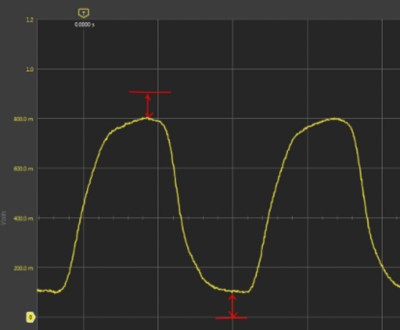Figure 2

## SDR, DDR, and 2X Edge Multiplier Examples

### DDR 1X (100 Mbps):

To demonstrate DDR, the example below will start with a double data rate interface running at 100 Mbps, with a vector period of 10 ns. The data would only need to change once every 10 ns, so it could simply be described with a NR pattern with the data and clock, with alternating 1’s and 0’s for the clock. Figures 3 and 4 show the timing sheet and pattern used, respectively, while Figure 4 shows the Digital Scope after bursting the pattern.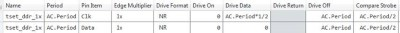Figure 3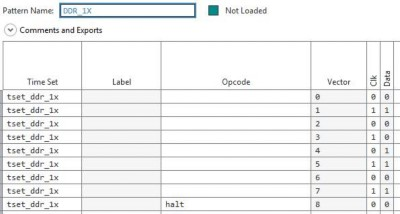Figure 4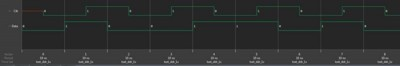Figure 5

### DDR 1X (200 Mbps):

However, if the goal is to run the DDR interface faster than 100 Mbps, you will need to change out timesets to describe the data, even though the digital pattern instrument might technically be capable of describing the waveform by using RL and RH formats. Depending on how many pins there are, this process could be difficult and confusing. The example below is a simple demonstration of this method. This method is not recommended. Instead the 2X edge multiplier feature should be used as shown in the DDR 2X (200 Mbps) section below.

Figure 7 depicts a new pattern demonstrating DDR, but it is being run at 200 Mbps without using the edge multiplier feature. As shown in Figure 6, the clock can be described using an RL format, which is simple. However, the return format for the data must be chosen based on the data to be transmitted. Figure 8 shows the Digital Scope after bursting the pattern.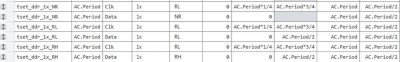Figure 6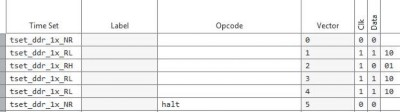Figure 7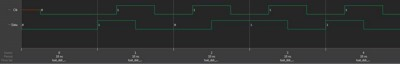Figure 8

So, while this is possible, it is confusing since it is necessary to change the format on a cycle by cycle basis. If there were more pins, then problems such as not having enough time sets become more likely. Additionally, if source memory was used, the digital pattern instrument would not have a way of generically supporting it, since the time sets cannot change based on the data.

### DDR 2X (200 Mbps):

The 2X edge multiplier feature was added to address this issue. It enables describing multiple data drive values within a single vector cycle. The same example from above is repeated here, this time using the 2X edge multiplier feature to make it much easier to describe. Figure 9 and 10 show the timing sheet and pattern used, respectively. Figure 11 shows the Digital Scope after bursting the pattern.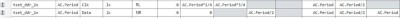Figure 9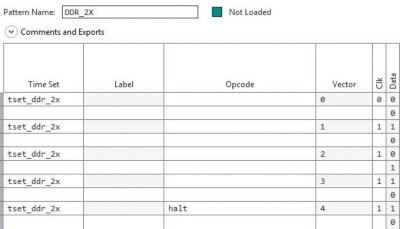Figure 10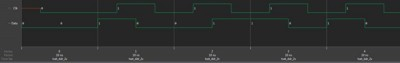Figure 11

### SDR 2X (133 Mbps):

While the same techniques could be used for getting the data to update at up to 200 Mbps, this would require a bit rate on the clock of 400 Mbps, which is too fast for the digital pattern instrument (unless the consequences described above are acceptable for your application). With a minimum pulse width of 3.75 ns, the best the digital pattern instrument can do is a clock bit rate of 266 Mbps, which is a 133 MHz clock and 133 Mbps data. Figure 12 shows the pattern and Figure 13 shows the Digital Scope after bursting the pattern. Note that the period used in this example changed from 10 ns to 15 ns in order to fit timing requirements.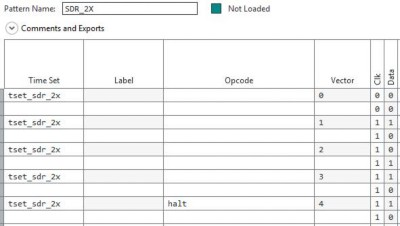Figure 12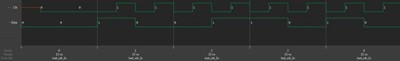Figure 13